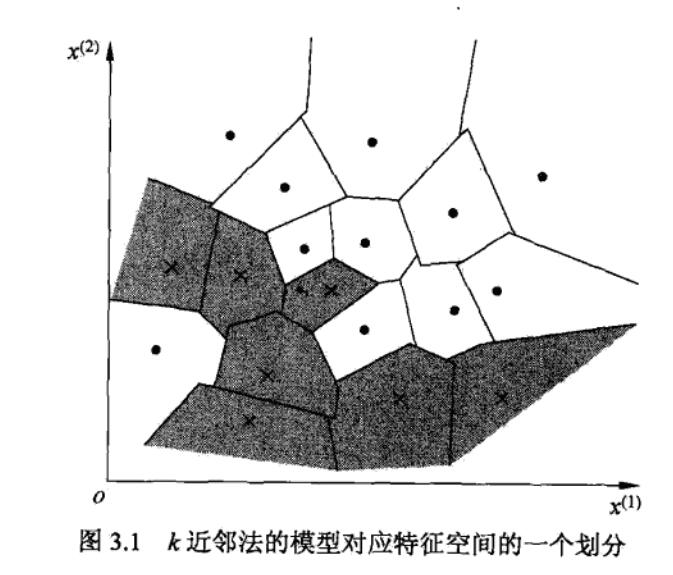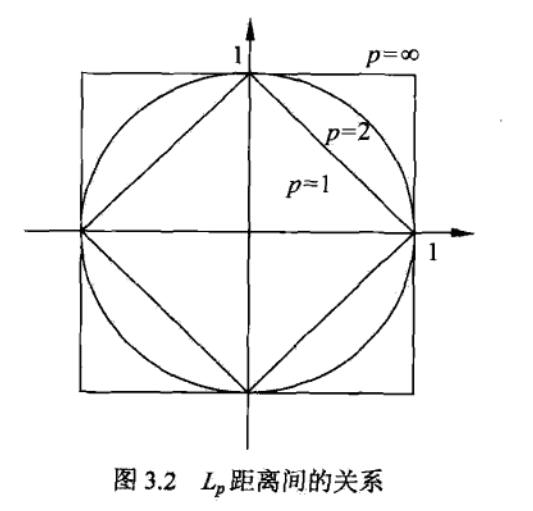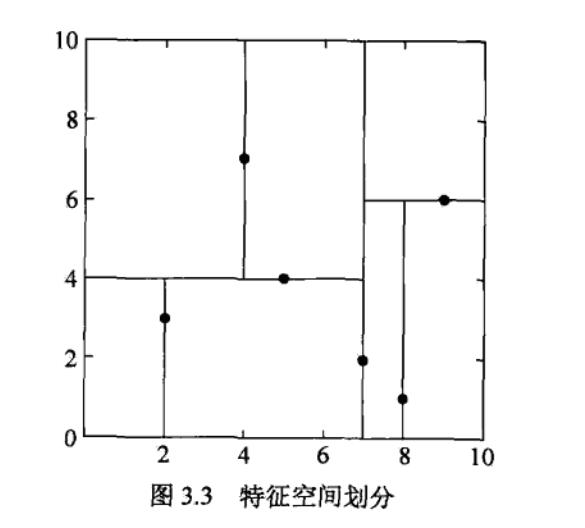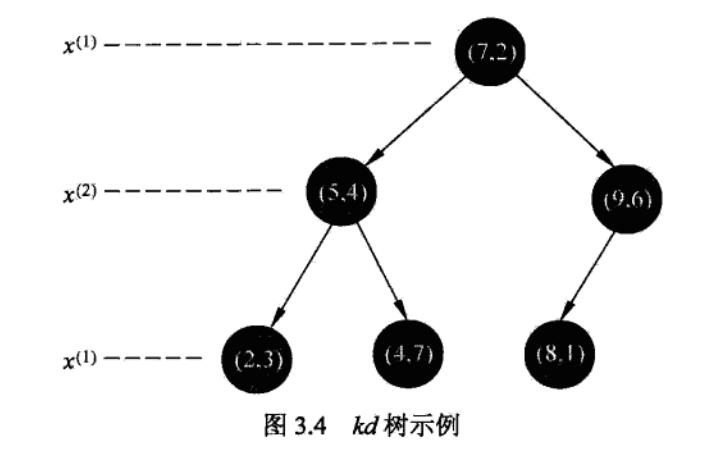# 李航-统计学习方法-笔记-3：KNN

## KNN算法

KNN没有显式的学习过程。

KNN使用的模型实际上对应于特征空间的划分。特征空间中，对每个训练实例点$$x_i$$，距离该点比其它点更近的所有点组成一个区域，叫作单元（cell）。每个训练实例拥有一个单元。所有的训练实例点的单元构成对特征空间的一个划分。如下图所示。## KNN三要素之1——距离度量

KNN的特征空间一般是n维实数向量空间$$R^n$$，使用的距离是欧氏距离。但也可以是其他距离，如更一般的$$L_p$$距离。设$$x_i$$为第$$i$$个样本，$$n$$维向量，$$x_i = (x_i^{(1)}, x_i^{(2)}, ..., x_i^{(n)})^T$$，则

$L_p(x_i, x_j) = (\sum_{l=1}^{n} |\ x_i^{(l)} - x_j^{(l)}\ |^{\ p})^{\frac{1}{p}}, p \geqslant 1 \\ L_\infty(x_i, x_j) = \max_{l} |x_i^{(l)} - x_j^{(l)}|$

$$p=2$$时称为欧式距离，$$p=1$$称为曼哈顿距离。下图是二维空间中，与原点的$$L_p$$距离为1的图形。

$$p=1$$时，$$|x| + |y| = 1$$
$$p=2$$时，$$x^2 + y^2 = 1$$
$$p=\infty$$时，$$\max(|x|, |y|) = 1$$## KNN三要素之2——K值选择

K值的选择会对KNN结果产生重大影响

K值的减小意味着整体模型变得复杂，容易发生过拟合。

K值的增大意味着整体模型变得简单。K=N时，无论输入实例是什么，都将简单地预测它属于在训练实例中最多的类，这时模型过于简单，完全忽略训练实例中的大量有用信息，是不可取的。

K值一般取一个比较小的数值，通常采用交叉验证法来选取最优的K值。

## KNN三要素之3——分类决策规则

KNN的分类决策规则往往是多数表决，即由输入实例的K个邻近的训练实例中的多数类决定输入实例的类。

## KNN的实现：kd树

kd树：KNN最简单的实现方法是线性扫描。这时要计算输入实例与每一个训练实例的距离。当训练集很大时，计算非常耗时，这种方法是不可行的。

（1）开始：构造根结点，根结点对应于包含$$T$$的k维空间的超矩阵区域。

（2）重复：对深度为$$j$$的结点，选择$$x^{(j)}$$为切分的坐标轴，$$l = j(mod \ k) + 1$$，以该结点的区域中所有实例的$$x^{(l)}$$坐标的中位数为切分点。

（3）直到两个子区域没有实例存在时停止，从而形成kd树的区域划分。（1）从根结点出发，递归地向下访问kd树。若目标点x当前维的坐标小于切分点的坐标，则左移，否则右移，直到子结点为叶结点。
（2）以此叶结点为“当前最近点”。
（3）递归向上回退，在每个结点进行以下操作：

（a）若该结点保存的实例点比“当前最近点”离目标点更近，则以该实例点为“当前最近点”。

（b）检查另一子结点对应的区域是否与“以目标点为球心，以目标前与‘当前最近点’的距离为半径的超球体”相交。

（4）回退到根结点时，搜索结束。最后的“当前最近点”即为x的最近邻点。posted @ 2019-06-05 15:11  PilgrimHui  阅读(1363)  评论(0编辑  收藏  举报